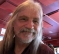## Quantizing the feedback coefficient in the Goertzel algorithmStarted by 6 months ago2 replieslatest reply 6 months ago153 views

The value of the feedback coefficient in the Goertzel algorithm it given by:

c = 2*cos( 2*pi*k/N);

where k is the bin number and N is the number of points.

Here Lyons shows that quantizing the coefficient to few bits does not affect the stability of the filter: it can be shown that the pole is guaranteed to lie on the unit circle no matter the number of bits used to represent the coefficient:

https://www.dsprelated.com/showarticle/796.php

At the same time, here the Goertzel algorithm is generalized for non-integer multiple of the fundamental frequency:

https://www.researchgate.net/publication/257879807...

Isn't the extra step needed for the generalization always required as soon as the coefficient is quantized? Of course quantizing to many bits likely pushes the error into don't care land, but curious about this in theory.

Thanks!

[ - ]It's the denominator coefficients, a1 and a2, that are getting quantized.

The specific frequency might be off, due to quantizing a1, but the radii of the pair of poles is the sqrt(a2).  When a2=1, you're on the unit circle and, no matter how wide your coefficient word is, you can always get a2=1.

[ - ]